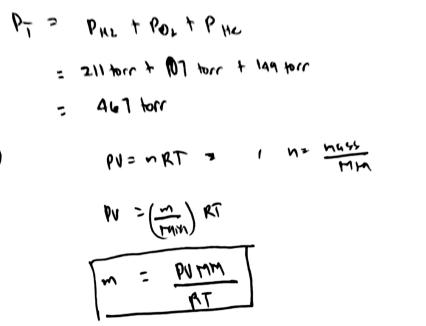# Problem: A gas mixture contains each of the following gases at the indicated partial pressures: N2, 211 torr; O2, 107 torr; and He, 149 torr. What is the total pressure in torr of the mixture? What mass in grams of each gas is present in a 1.15 - L sample of this mixture at 25.0 °C? Enter your answers numerically separated by commas.

###### FREE Expert Solution###### Problem Details

A gas mixture contains each of the following gases at the indicated partial pressures:

N2, 211 torr; O2, 107 torr; and He, 149 torr.

What is the total pressure in torr of the mixture?

What mass in grams of each gas is present in a 1.15 - L sample of this mixture at 25.0 °C?

What scientific concept do you need to know in order to solve this problem?

Our tutors have indicated that to solve this problem you will need to apply the Partial Pressure concept. You can view video lessons to learn Partial Pressure. Or if you need more Partial Pressure practice, you can also practice Partial Pressure practice problems.

What is the difficulty of this problem?

Our tutors rated the difficulty ofA gas mixture contains each of the following gases at the in...as medium difficulty.

How long does this problem take to solve?

Our expert Chemistry tutor, Dasha took 10 minutes and 5 seconds to solve this problem. You can follow their steps in the video explanation above.

What professor is this problem relevant for?

Based on our data, we think this problem is relevant for Professor Abraha's class at CNM.